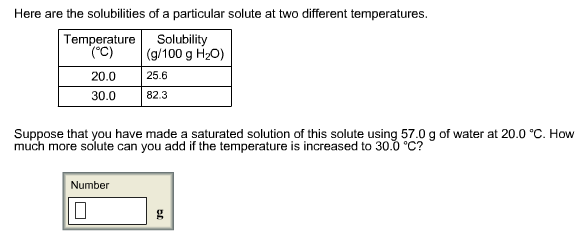# Problem: Here are the solubilities of a particular solute at two different temperatures. Suppose that you have made a saturated solution of this solute using 57.0 g of water at 20.0°C. How much more solute can you add if the temperature is increased to 30.0°C?

###### FREE Expert Solution
98% (109 ratings)###### Problem Details

Here are the solubilities of a particular solute at two different temperatures. Suppose that you have made a saturated solution of this solute using 57.0 g of water at 20.0°C. How much more solute can you add if the temperature is increased to 30.0°C?